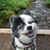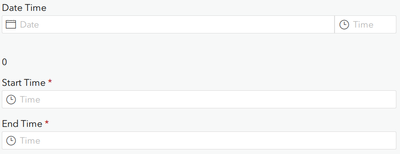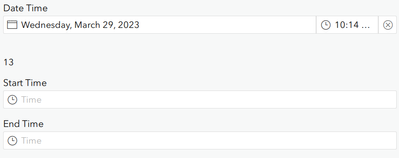# Required Conditional Questions Survey 123

169
3
03-29-2023 08:31 AMNew Contributor II

Hello! I am currently working on a compliant form, and often the complainant will not know the date or time of their visit but have a general idea or time frame.

At the moment, I have a date and time question and start time and end time, IF the date and time question are left NULL, I want the survey to required the end user to fill out the start and end time BUT only if the date and time question are null. Is this possible?

Tags (2)
1 Solution

Accepted Solutionsby
New Contributor III

Hey there,

If I'm understanding it right, you have a dateTime question along with a time question for Start Time and another time question for End Time. You want the two Time questions to be required IF the dateTime question is not filled?

If so, this can definitely be achieved by converting the dateTime question to a string and setting a formula in the required column for the time questions.

If you convert the dasteTime question to a string, when it's blank the value it returns is 0. Thus, we want to set the required condition for the time questions to only be required if the string-length of the dateTime question is 0.

In the "required" column for the two time questions, use the following formula;

string-length(\${<dateTime question name>}) = 0

This makes the start and and time questions required when dateTime is blank.But not required when dateTime is filledHope I understood that right! If that needs to be altered for dateTime questions as opposed to just time questions, the same required calculation should still be valid.

3 Repliesby
New Contributor III

Hey there,

If I'm understanding it right, you have a dateTime question along with a time question for Start Time and another time question for End Time. You want the two Time questions to be required IF the dateTime question is not filled?

If so, this can definitely be achieved by converting the dateTime question to a string and setting a formula in the required column for the time questions.

If you convert the dasteTime question to a string, when it's blank the value it returns is 0. Thus, we want to set the required condition for the time questions to only be required if the string-length of the dateTime question is 0.

In the "required" column for the two time questions, use the following formula;

string-length(\${<dateTime question name>}) = 0

This makes the start and and time questions required when dateTime is blank.But not required when dateTime is filledHope I understood that right! If that needs to be altered for dateTime questions as opposed to just time questions, the same required calculation should still be valid.New Contributor II

@Vinzafy thank you so much. This is exactly what I needed.by
New Contributor III

Fantastic! Glad that worked for you 🙂